# 发现一个宝藏 Python 库,玩社区发现算法的不能错过!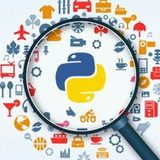日常学python |27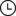2022-11-28 00:34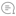0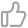00

## 推荐阅读： 终于来了，【第三期】 彭涛Python 爬虫特训营！!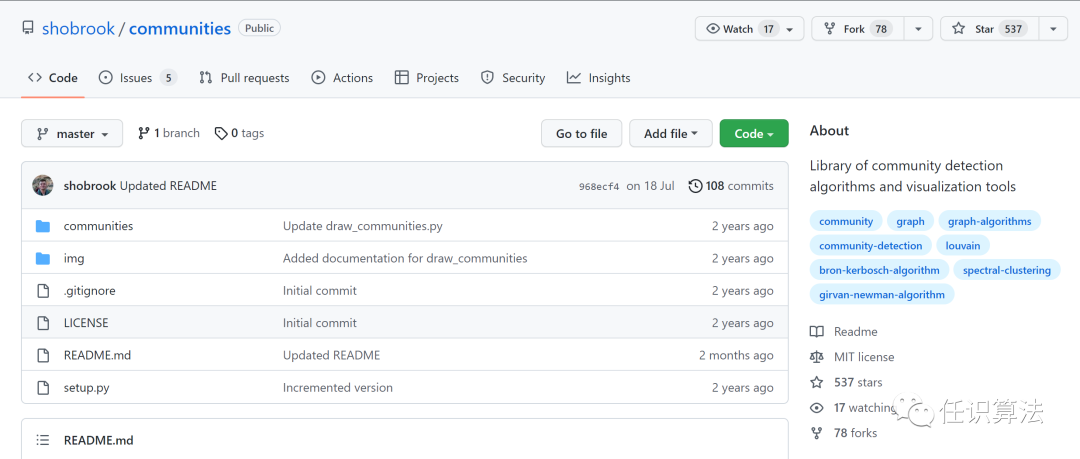• Louvain 算法
• Girvan-Newman 算法
• 层次聚类
• 谱聚类
• Bron-Kerbosch 算法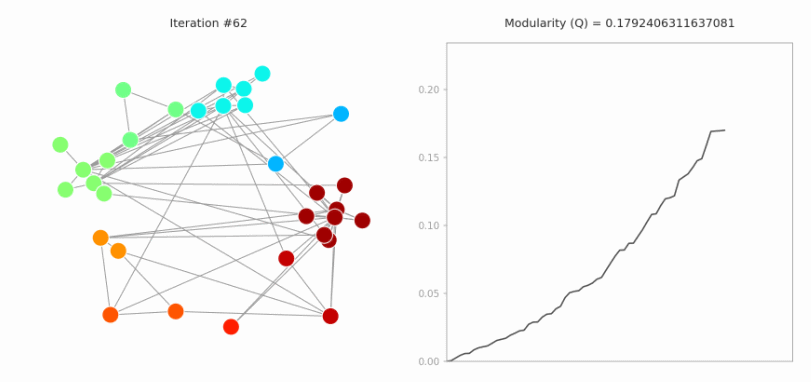图片

```      `import numpy as npfrom communities.algorithms import louvain_methodadj_matrix = np.array([[0, 1, 1, 0, 0, 0],                       [1, 0, 1, 0, 0, 0],                        [1, 1, 0, 1, 0, 0],                        [0, 0, 1, 0, 1, 1],                          [0, 0, 0, 1, 0, 1],                       [0, 0, 0, 1, 1, 0]])communities, _ = louvain_method(adj_matrix)>> communities[{0, 1, 2}, {3, 4, 5}]`
```图片

1、Louvain 算法

```      `louvain_method(adj_matrix : numpy.ndarray, n : int = None) -> list`
```

Louvain 算法对最大化图模块性的社区进行贪婪搜索。如果一个图具有高密度的群体内边缘和低密度的群体间边缘，则称之为模图。

```      `from communities.algorithms import louvain_methodadj_matrix = [...]communities, _ = louvain_method(adj_matrix)`
```

2、Girvan-Newman 算法

```      `girvan_newman(adj_matrix : numpy.ndarray, n : int = None) -> list`
```

Girvan-Newman 算法迭代删除边以创建更多连接的组件。每个组件都被视为一个 community，当模块度不能再增加时，算法停止去除边缘。

```      `from communities.algorithms import girvan_newmanadj_matrix = [...]communities, _ = girvan_newman(adj_matrix)`
```

3、层次聚类

```      `hierarchical_clustering(adj_matrix : numpy.ndarray, metric : str = "cosine", linkage : str = "single", n : int = None) -> list`
```

```      `from communities.algorithms import hierarchical_clusteringadj_matrix = [...]communities = hierarchical_clustering(adj_matrix, metric="euclidean", linkage="complete")`
```

4、谱聚类

```      `spectral_clustering(adj_matrix : numpy.ndarray, k : int) -> list`
```

```      `from communities.algorithms import spectral_clusteringadj_matrix = [...]communities = spectral_clustering(adj_matrix, k=5)`
```

5、Bron-Kerbosch 算法

```      `bron_kerbosch(adj_matrix : numpy.ndarray, pivot : bool = False) -> list`
```
Bron-Kerbosch 算法实现用于最大团检测（maximal clique detection）。图中的最大团是形成一个完整图的节点子集，如果向该子集中添加其他节点，则它将不再完整。将最大团视为社区是合理的，因为团是图中连接最紧密的节点群。因为一个节点可以是多个社区的成员，所以该算法有时会识别重叠的社区。 示例代码如下：
```      `from communities.algorithms import bron_kerboschadj_matrix = [...]communities = bron_kerbosch(adj_matrix, pivot=True)`
```

```      `draw_communities(adj_matrix : numpy.ndarray, communities : list, dark : bool = False, filename : str = None, seed : int = 1)`
```

```      `from communities.algorithms import louvain_methodfrom communities.visualization import draw_communitiesadj_matrix = [...]communities, frames = louvain_method(adj_matrix)draw_communities(adj_matrix, communities)`
```Louvain 算法的动图展示

```      `louvain_animation(adj_matrix : numpy.ndarray, frames : list, dark : bool = False, duration : int = 15, filename : str = None, dpi : int = None, seed : int = 2)`
```

Louvain 算法在图中的应用可以实现动图展示，其中每个节点的颜色代表其所属的社区，并且同一社区中的节点聚类结合在一起。

```      `from communities.algorithms import louvain_methodfrom communities.visualization import louvain_animationadj_matrix = [...]communities, frames = louvain_method(adj_matrix)louvain_animation(adj_matrix, frames)`
```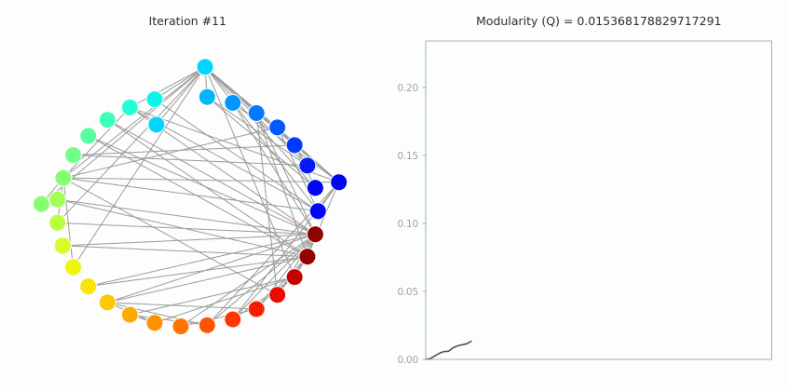图片

https://www.codenong.com/cs105912940/

···  END  ···

```
```
```
```2个企业实战项目，4大常用工具00

暂无评论~~
Ctrl+Enter